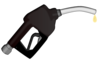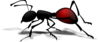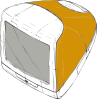Click here for the Problem Extension Worksheet version of the Problems of the Week.
Click here for an MS Word version of the Problems of the Week.
Click here for the Canadian Problem Extension Worksheet version of the Problems of the Week.
Click here for a Canadian MS Word version of the Problems of the Week.Lower Elementary:
Question: Derek has won 3 awards. Hansel has won 1 award. What fraction of all the awards did Derek win?
Solution: To find the fraction, we first need to add up all of the awards to find the whole: 3 + 1 = 4. Derek won 3 out of the 4 awards, so the fraction of all the awards that Derek won is 3/4.Upper Elementary:
Question: Gasoline costs \$1.45 per gallon. If a car’s gas tank holds 15 gallons in total and is only 1/5 full right now, then how much will it cost to fill up the tank?
Solution: If the gas tank is 1/5 full, then it will need 4/5 × 15 = 12 gallons of gas to fill it. The 12 gallons of gas it takes to fill the tank costs \$1.45 × 12 = \$17.40.Middle School:
Question: An ant is 1/16 of an inch tall. A middle school student is 41/2 feet tall. The student goes to a learning center that is 54 feet tall. If we scale by height, then how tall would a learning center for ants be?
Answer: 3/4 of an inch
Solution: The learning center is 54 ÷ 41/2 = 12 times the height of the student. So, the learning center for ants should be 12 times the height of the ant. Since 1/16 × 12 = 12/16 and 12/16 reduces to 3/4, the learning center for ants would be 3/4 of an inch tall.Algebra and Up:
Question: A man throws a computer off of a platform straight to the ground. It falls 16 feet and hits the ground in 1/2 of a second. Consider this equation for vertical distance traveled in terms of initial velocity, time, and acceleration due to gravity (32 feet/second2):

(distance) = (initial velocity)(time) – 1/2(acceleration due to gravity)(time)2
What is the initial velocity of the computer?
(Hint: The computer is traveling straight down, so the distance it travels is -16 feet in this instance.)
Answer: 24 feet per second straight down
Solution: If we plug all our given values into the equation, we get this:

-16 = x(1/2) – 1/2(32)( 1/2)2
If we simplify that equation, we get this:

-16 = x(1/2) – 4
If we then solve for x, we get -24. The initial velocity of the computer is therefore 24 feet per second straight down.